Computer Science

# Lab 7 - Functions

## What Does It Print?

def foo():
print("I love")
print("to program")

foo()
foo()
def foo(number):
print(f"The numbers is: {number}")

foo(7)
foo(10)
def foo(word):
print(word)
print(word + 7)

foo(3)
foo(5)
def bar(number):
print(number + 2)

def foo(number):
bar(number)
bar(number + 3)

foo(2)
foo(8)
def baz(number):
print(number - 1)

def bar(number):
print(number)
baz(number)

def foo(number):
bar(number + 2)
baz(number)

foo(7)
foo(4)

## What Does It Draw?

### Blue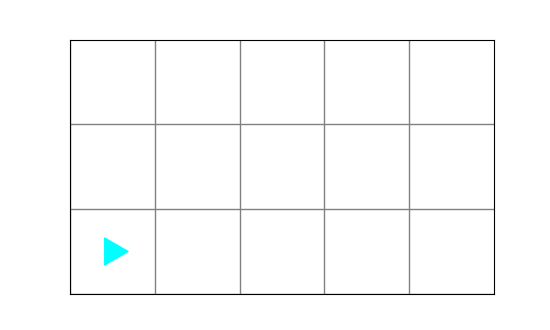from byubit import Bit

def go_blue(bit):
while bit.front_clear():
bit.paint('blue')
bit.move()

@Bit.run_from_empty(5, 3)
def go(bit):
go_blue(bit)
bit.left()
bit.move()
bit.left()
bit.move()
go_blue(bit)
Results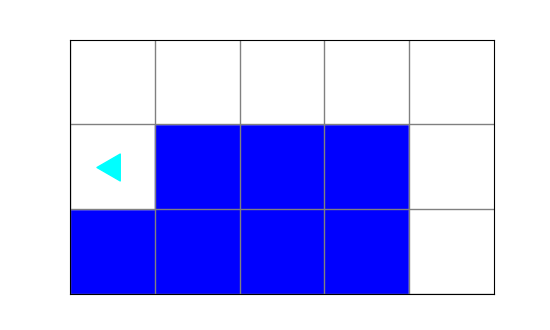### Red Stuff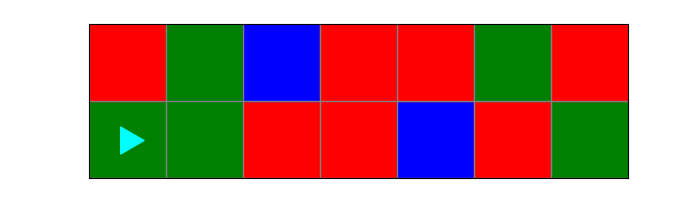from byubit import Bit

def fix_red(bit):
while bit.front_clear():
if bit.get_color() == 'red':
bit.paint('blue')
bit.move()

@Bit.run('red-stuff')
def go(bit):
fix_red(bit)
bit.left()
bit.move()
bit.left()
fix_red(bit)
Results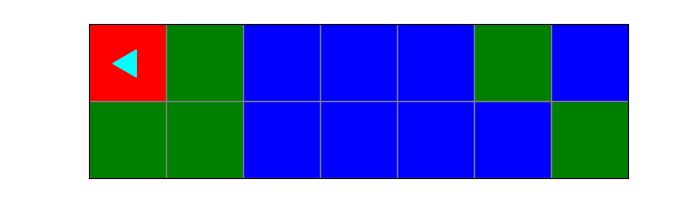### Leaping

from byubit import Bit

def leap(bit):
if bit.front_clear():
bit.move()
if bit.front_clear():
bit.move()
if bit.front_clear():
bit.move()

def paint_spots(bit):
while bit.front_clear():
bit.paint('blue')
leap(bit)
bit.paint('blue')

def fill_green(bit):
while bit.front_clear():
bit.paint('green')
bit.move()
bit.paint('green')

@Bit.run_from_empty(6, 3)
def go(bit):
fill_green(bit)
bit.left()
bit.left()
paint_spots(bit)

Results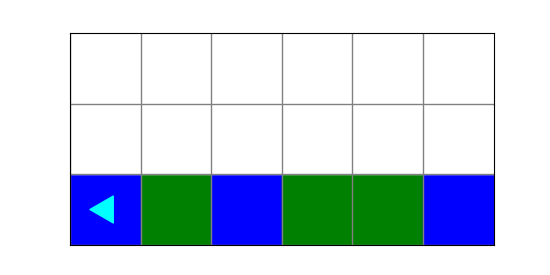## Activities

### smile.py

Draw smiles on each of the green squares.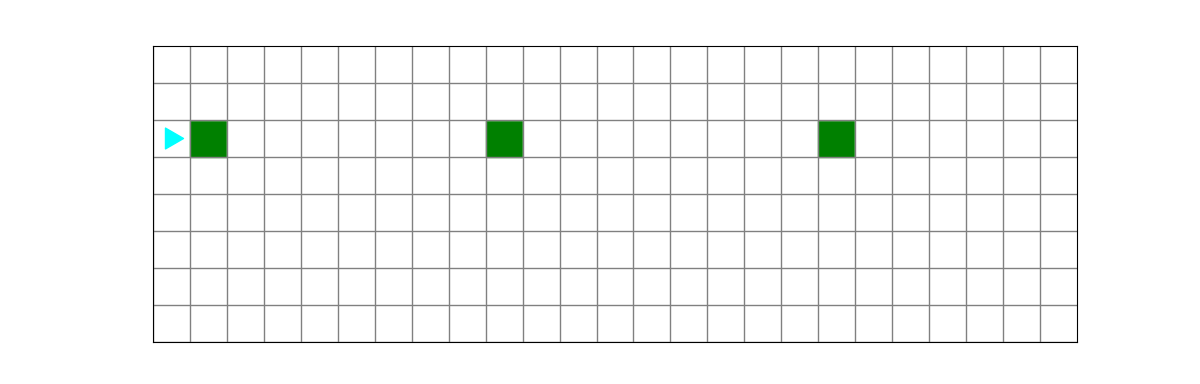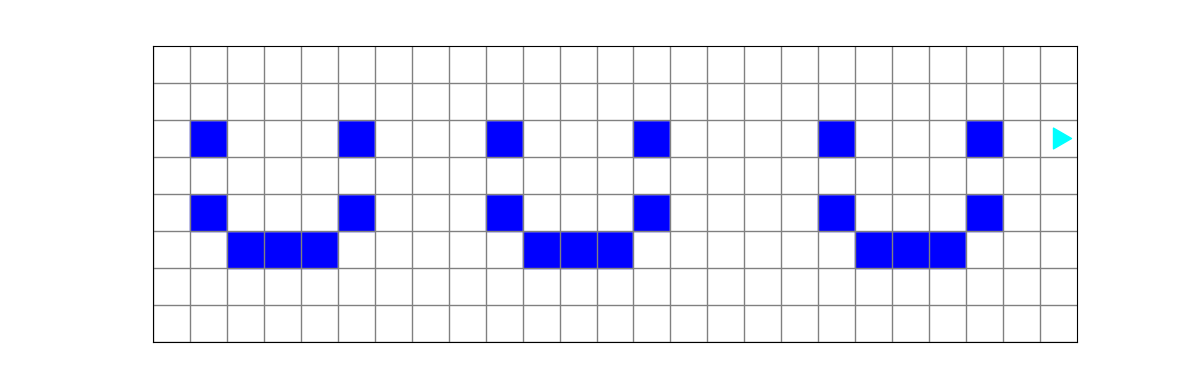### fix_forest.py

Fix all the trees in the forest.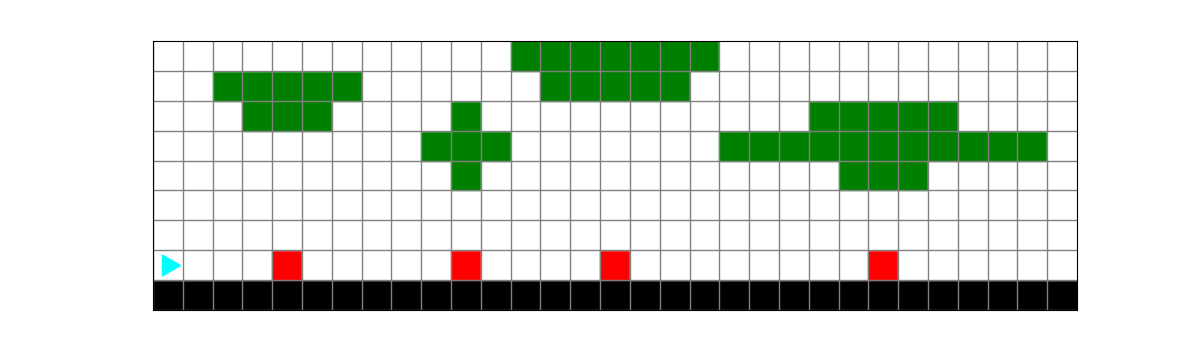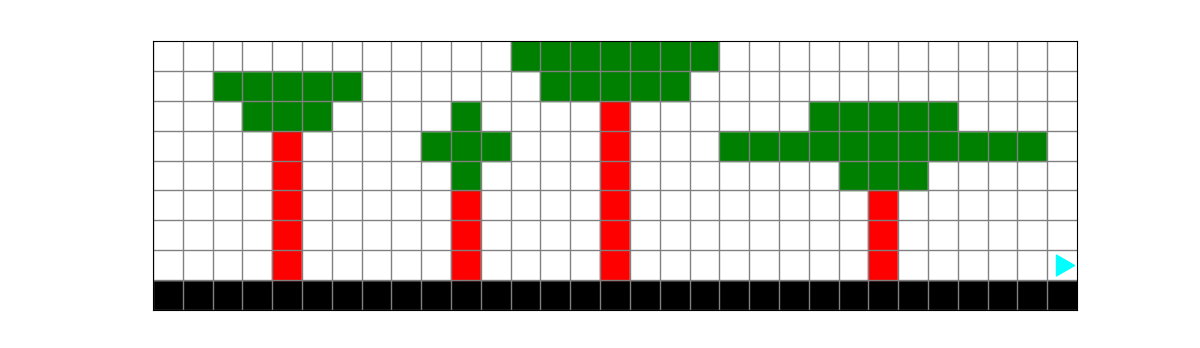### surround.py

Surround each block block with green squares, but leave the corners empty.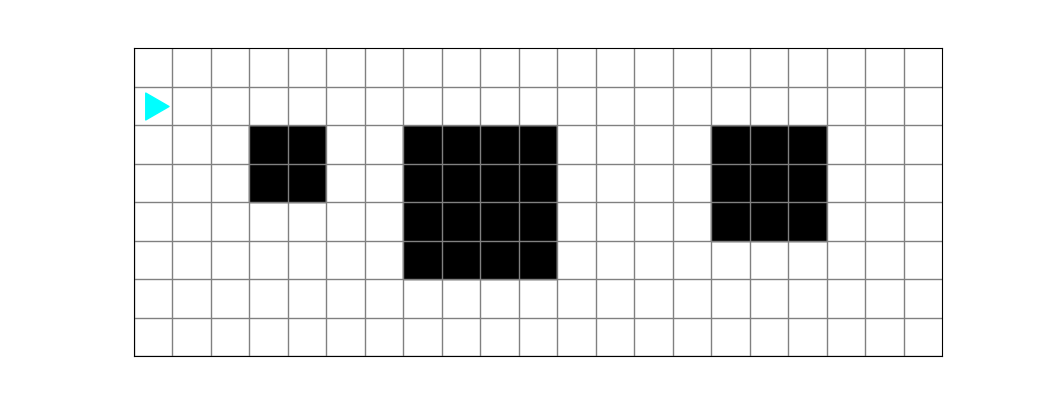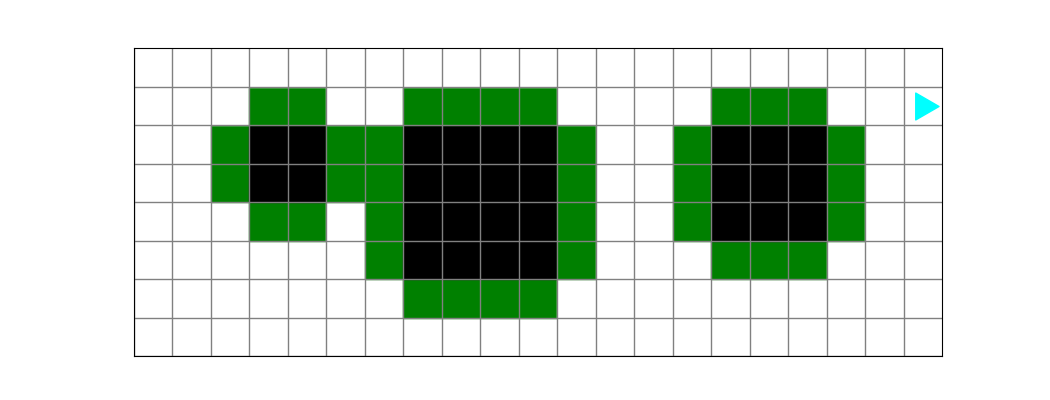### blossoms.py

The desert is dry. Fill in the holes with water and add flowers next to each pool.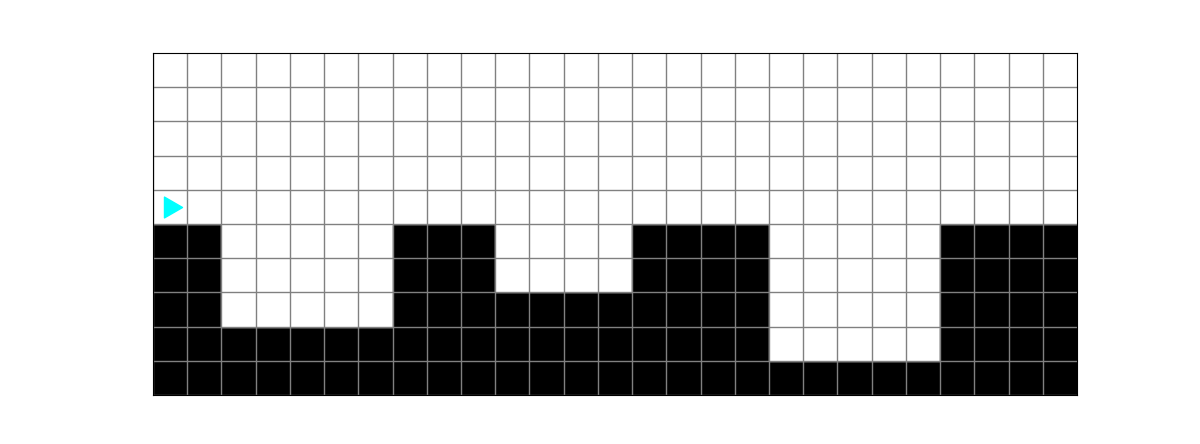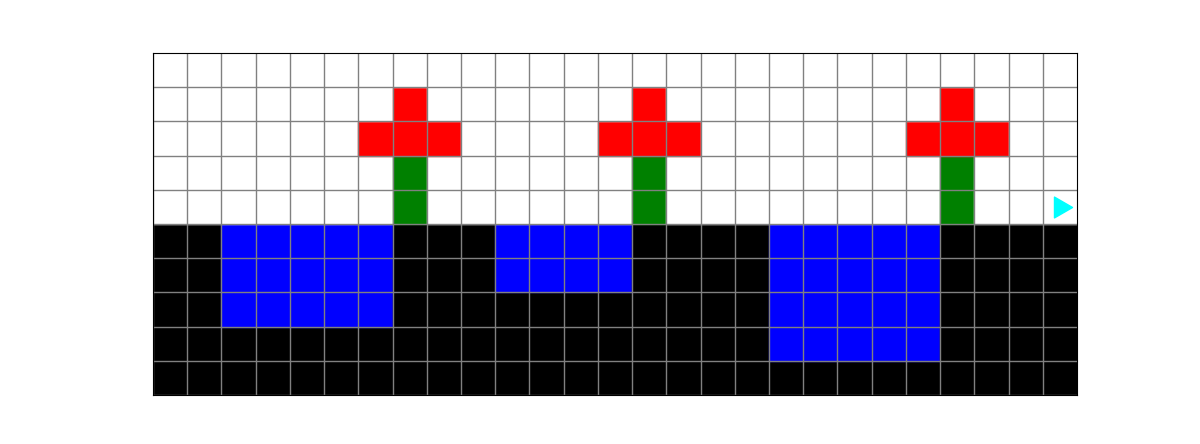### soccer_fields.py

The soccer fields need grass!

Each field is connected to the next field by a small exit.

Fill each field with grass.

Note: The exit from one field to the next will never be in the top row of the field.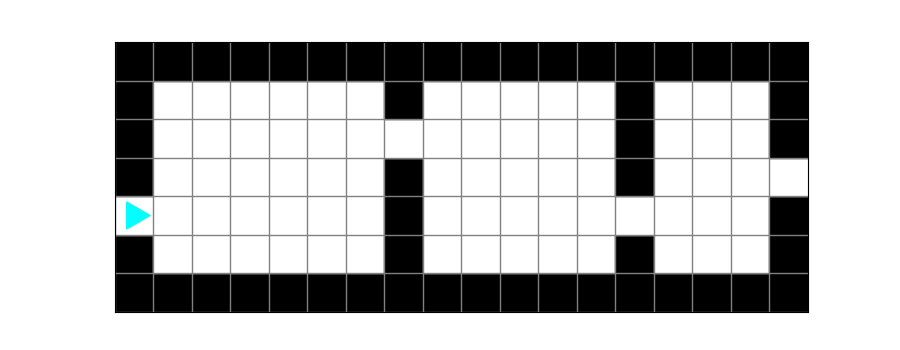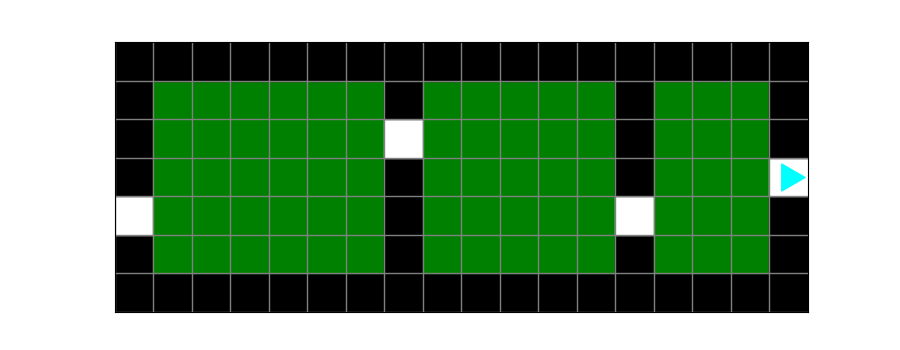### barcode.py

Finish the barcodes by extending the blue lines to the top of the grid.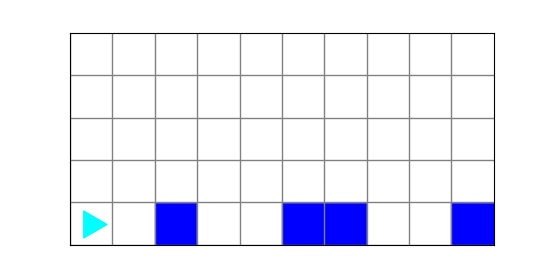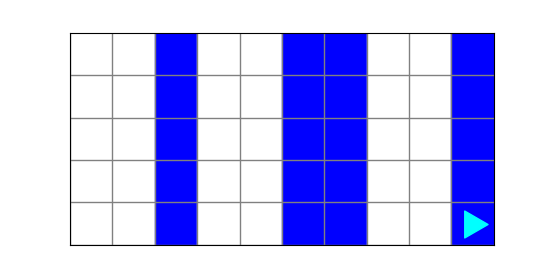smile.py5
fix_forest.py5
surround.py5
blossoms.py5
soccer_fields.py5
barcodes.py5